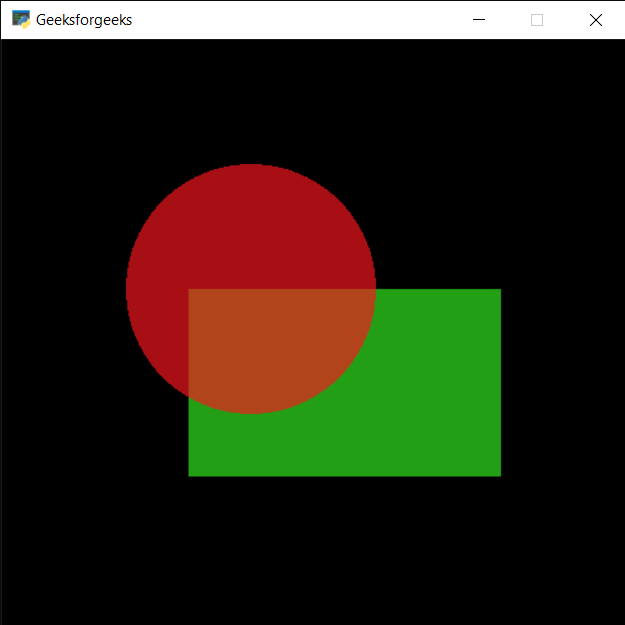# PYGLET – Shape Opacity

• Last Updated : 18 Aug, 2021

In this article, we will see how we can access the opacity the shape in PYGLET module in python. Pyglet is easy to use but powerful library for developing visually rich GUI applications like games, multimedia, etc. A window is a “heavyweight” object occupying operating system resources. Windows may appear as floating regions or can be set to fill an entire screen (fullscreen). A shape is the form of an object or its external boundary, outline, or external surface, as opposed to other properties such as color, texture or material type. Many shapes are drawn with the help of shapes module of pyglet. Opacity is the measure of impenetrability to electromagnetic or other kinds of radiation, especially visible light. In radiative transfer, it describes the absorption and scattering of radiation in a medium, such as a plasma, dielectric, shielding material, glass, etc.

We can create a window with the help of the command given below

```# creating a window
window = pyglet.window.Window(width, height, title)```

In order to create window we use opacity attribute with the shape
Syntax : shape.opacity
Argument : It takes no argument
Return : It returns integer

Below is the implementation

## Python3

 `# importing pyglet module``import` `pyglet` `# importing shapes from the pyglet``from` `pyglet ``import` `shapes` `# width of window``width ``=` `500``  ` `# height of window``height ``=` `500``  ` `# caption i.e title of the window``title ``=` `"Geeksforgeeks"``  ` `# creating a window``window ``=` `pyglet.window.Window(width, height, title)` `# creating a batch object``batch ``=` `pyglet.graphics.Batch()`  `# properties of rectangle``# co-ordinates of rectangle``co_x ``=` `150``co_y ``=` `150` `# width of rectangle``width ``=` `250` `# height of rectangle``height ``=` `150` `# color = green``color ``=` `(``50``, ``225``, ``30``)` `# creating a rectangle``rec ``=` `shapes.Rectangle(co_x, co_y, width, height, color ``=` `color, batch ``=` `batch)` `# changing opacity of the rect1``# opacity is visibility (0 = invisible, 255 means visible)``rec.opacity ``=` `180`  `# creating another rectangle with properties``# x, y co ordinate : 50, 250``# width, height of rectangle : 300, 200``# color = red``color ``=` `(``255``, ``25``, ``25``)` `# properties of circle``# co-ordinates of circle``circle_x ``=` `200``circle_y ``=` `300` `# size of circle``# color = green``size_circle ``=` `100` `# creating a circle``circle ``=` `shapes.Circle(circle_x, circle_y, size_circle, color ``=``(``250``, ``22``, ``30``), batch ``=` `batch)` `# changing opacity of the circle1``# opacity is visibility (0 = invisible, 255 means visible)``circle.opacity ``=` `170`  `# window draw event``@window``.event``def` `on_draw():``    ` `    ``# clear the window``    ``window.clear()``    ` `    ``# draw the batch``    ``batch.draw()``    `  `# accessing opacity of rectangle``value_rec ``=` `rec.opacity` `# printing value``print``(``"Rectangle : "``, end ``=` `"")``print``(value_rec)` `# accessing opacity of circle``value_cir ``=` `circle.opacity` `# printing value``print``(``"Circle : "``, end ``=` `"")``print``(value_cir)`   `# run the pyglet application``pyglet.app.run()`

Output :```Rectangle : 180
Circle : 170```

My Personal Notes arrow_drop_up